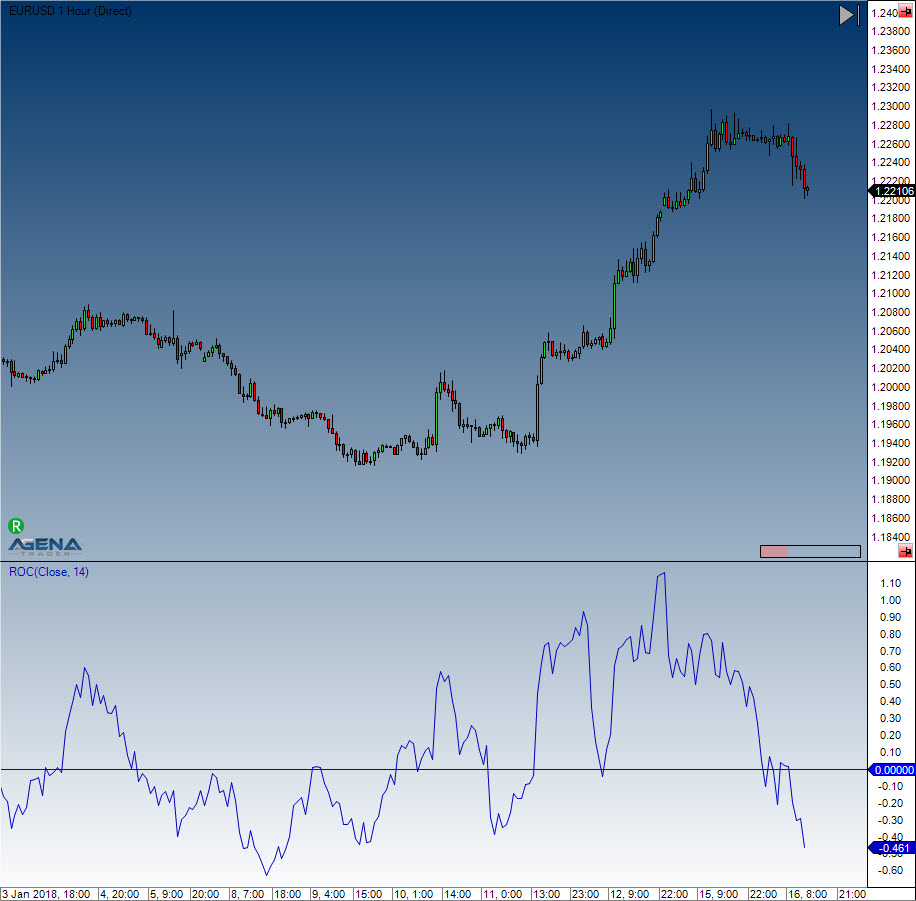# Rate of Change (ROC)

#### Description

The Rate of Change Indicator is a simple yet effective momentum oscillator. It measures the relative percentage change of the prices from one period to the next. The calculation looks at the current price and compares it to the price of n periods ago.

#### Interpretation

As long as the ROC is above 0, the uptrend is intact. As long as the ROC is below 0, the downtrend is intact. Divergences between the indicator and the price development can hint to a trend change.

#### Usage

ROC(int period)
ROC(IDataSeries inSeries, int period)
ROC(int period)[int barsAgo]
ROC(IDataSeries inSeries, int period)[int barsAgo]

#### Return value

double
When using this method with an index (e.g. ROC(14)[int barsAgo] ), the value of the indicator will be issued for the referenced bar.

#### Parameters

inSeries Input data series for the indicator
period Number of bars included in the calculations

#### VisualizationRate of Change (ROC)